# KSEEB Solutions for Class 7 Maths Chapter 11 Perimeter and Area Ex 11.3

Students can Download Chapter 11 Perimeter and Area Ex 11.3, Question and Answers, Notes Pdf, KSEEB Solutions for Class 7 Maths, Karnataka State Board Solutions help you to revise complete Syllabus and score more marks in your examinations.

## Karnataka State Syllabus Class 7 Maths Chapter 11 Perimeter and Area Ex 11.3

Question 1.
Find the circumference of the circles with the following radius :
(Take n = $$\frac{22}{7}$$)
a) 14 cm
radius = r = 14 cm
∴ circumference of a circle = 2πr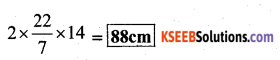b) 28 mm
radius = r = 28 cm
∴ circumference of a circle = 2πr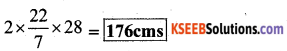c) 21 cm
radius = r = 21 cm
circumference of a circle = 2πr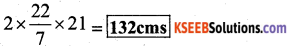Question 2.
Find the area of the following circles, given that:

a) radius = 14 mm (Take π = $$\frac{22}{7}$$)
∴ Area of the circle = πr2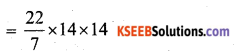= 616 sq.cms

b) diameter = 49 m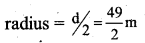Area of the circle = πr2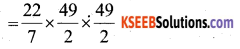= 1,886.5 sq.metrs

radius = r = 5 cm
∴ Area of the circle = πr2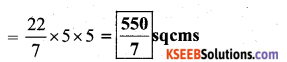Question 3.
If the circumference of a circular sheet is 154m, find its radius. Also find the area of the sheet. (Take π = $$\frac{22}{7}$$)
Solution:
C = circumference of a circle = 154m = 2πr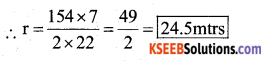Area of the sheet = πr2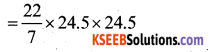= 1,886.5 sq.metrsQuestion 4.
A gardener wants to fence a circular garden of diameter 21m. Find the length of the rope he needs to purchase, if he makes 2 rounds of fence. Also find the cost of the rope, if it costs ₹ 4 per meter.
(Take π = $$\frac{22}{7}$$)
d = diameter = 21 m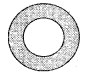∴ radius of the circular garden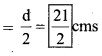circumference of the circular garden = 2πr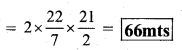Length of the rope needed to make one round of fence } = 66 mts
Length of the rope needed to make 2 rounds =
66 × 2 = 132 mts
cost of rope per metre = Rs. 4
Total cost of rope = 132 × 4 = Rs. 528

Question 5.
From a circular sheet of radius 4 cm, a circle of radius 3 cm is removed. Find the area of the remaining sheet.
(Take π = 3.14)
Solution:
Radius of the outer circle = 4 cms.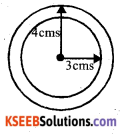∴ Area of the outer circle = πr2
= 3.14 × 4 × 4
= 28.26 sq.cms
= 50.24 sq.cms
π = 3.14
Radius of the inner circle = 3 cms
∴ Area of the inner circle = πr2
= 3.14 × 3 × 3 = 28.26 sq. cms
Area of the remaining part = 50.24 – 28.26 = 21.98 sq.cmsQuestion 6.
Saima wants to put lace on the edge of a circular table cover of diameter 1.5m. Find the length of the lace required and also find its cost if one meter of the lace
costs ₹ 15. (Take π = 3.14)
Solution:
Diameter of the table cover = 1.5m
Circumference of the table cover }
2πr – πd = 3.14 × 1.5 (∵ d = 2r) = 4.710 mts
∴ Length of the lace required = 4.71 mt
Cost of lace per meter = Rs. 15
∴ Total cost of the lace =4.71 × 15 = Rs 70.65

Question 7.
Find the perimeter of the adjoining figure, which is a semicircle including its diameter.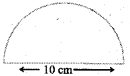Solution:
Diameter of the semi-circle = 10 cm
Circumference of semi-circle =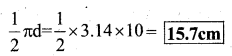Perimeter of the semi-circle(including its diameter) } = 15.7 + 10 = 25.7 cmsQuestion 8.
Find the cost of polishing a circular table-top of diameter 1.6m, if the rate of polishing is ? 15/m2. (Take π = 3.14)
Solution:
Diameter of the Table Top = 1.6 m
Area of the Table Top = πr2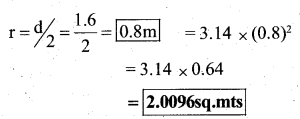Rate of polishing = Rs. 15/sq.m
Total cost of polishing = 2.0096 × 15
= Rs.30.14Question 9.
Shazil took a wire of length 44 cm and bent it into the shape of a circle. Find the radius of that circle. Also find its area. If the same wire is bent into the shape of a square, what will be the length of each of its sides ? Which figure encloses more area, the circle or the square? (Take π = $$\frac{22}{7}$$)
Solution:
Length of the wire = 44 cms
Total length of the circle = perimeter of the circle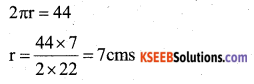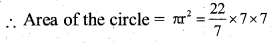∴ Length of wire = perimeter of the square = 154 sq.cms
44 = 44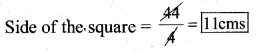Area of the square = a2 = 11 × 11 = 121 sq.cms.
∴ The area of the circle is more means the circle encloses more area.

Question 10.
From a circular card sheet of radius 14cm, two circles of radius 3.5 cm and a rectangle of length 3 cm and breadth 1 cm are removed, (as shown in the adjoining figure). Find the area of the remaining sheet. (Take π = $$\frac{22}{7}$$)
Solution:
Radius of the circular Card = 14 cm
Area of the Card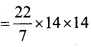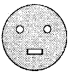Area of small 2 circles =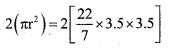= 77 sq. cms.
Area of rectangle = l × b = 3 × 1 = 3 sq. cms
Area of the remaining sheet
= 616 – (77 + 3)
= 616 = 80 = 536 sq.cmQuestion 11.
A circle of radius 2 cm is cut out from a square piece of an aluminium sheet of side 6 cm. What is the area of the leftover aluminium sheet? (Take π = 3.14)
Solution:
Area of square aluminium sheet = 6 × 6 = 36 sq. cms
Side = 6 cm radius = 2 cm
Area of circle =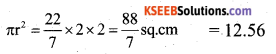Area of the left over Aluminium sheet = 36 – 12.56 = 23.44sq.cm

Question 12.
The circumference of a circle is 31.4. Find the radius and the area of the circle? (Take π = 3.14)
Solution:
The circumference of a circle = 2πr = 31.4 cm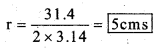Area of the cirlce = πr2
= 3.14 × 5 × 5
= 78.5 sq.cms

Question 13.
A circular flower bed is surrounded by a path 4 m wide. The diameter of the flower bed is 66m. What is the area of this path? (π = 3.14)
Solution: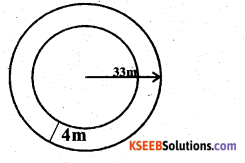Diameter of the flower bed = 66 m
Radius = 66/2 = 33 m
Area of the flower bed = πr2
= 3.14 × 33 × 33
= 3419.46 sq.cm
Radius of the flower bed with path = 33 + 4 = 37 ms.
Area of the flower bed with path =
= 3.14 × 37 × 37
= 4298.66 sq.cm
Area of the path = Area of the flower bed with path – Area of the flower bed
= 4298.66 – 3419.46
= 879.2 sq.mtsQuestion 14.
A circular flower garden has an area of 314 m2. A sprinkler at the centre of the garden can cover an area that has a radius of 12 m. Will sprinkler water the
entire garden? (Take π = 3.14)
Solution:
Area of the circular flower garden = 314 sq.m
Radius of the sprinkler = 12m
Area of the sprinkler = πr2 = 3.14 × 12 × 12
= 452.16 sq.mt
∴ 452.16 > 314
∴ Yes, the sprinkler water the entire garden.

Question 15.
Find the circumference of the inner and the outer circles, shown in the adjoining figure? (Take π = 3.14)
Solution: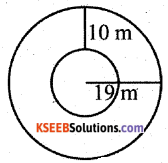Circumference of the outer circle = 2πr
= 2 × 3.14 × 19
= 119.32 mts
Circumference of the inner circle = 2πr
radius = 19 – 10 = 9 cm.
= 2 × 3.14 × 9
= 56.52 cm

Question 16.
How many times a wheel of radius 25cm must to go 352 m ? (Take π = $$\frac{22}{7}$$)
Solution:
radius of the wheel = r = 28 cm
circumference of the wheel = 2πr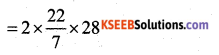= 176 cms
To cover the distance of 352 mtrs (∵ 1 m = 100 cm)
No. of rotation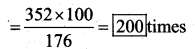Question 17.
The minute hand of a circular clock is 15 cm long. How far does the tip of the minute hand move in 1 hour? (Take π = 3.14)
Solution:
Length of the minute hand = 15 cm
1 hour = 1 round = circumference = 2πr
= 2 × 3.14 × 15
= 94.2 cms
∴ The tip of the minute hand in one hour moves 94.2 cms.